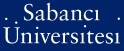# Unconditional convergence of spectral decompositions of 1D Dirac operators with regular boundary conditions

Djakov, Plamen Borissov and Mityagin, Boris Samuel (2012) Unconditional convergence of spectral decompositions of 1D Dirac operators with regular boundary conditions. Indiana University Mathematics Journal, 61 (1). pp. 359-398. ISSN 0022-2518

Full text not available from this repository.

Official URL: http://dx.doi.org/10.1512/iumj.2012.61.4531

## Abstract

One-dimensional Dirac operators L-bc(v)y = i(1 0 0 -1) dy/dx + v(x)y, y = (y(1)y(2)), x is an element of [0, pi], considered with L-2-potentials v (x) = (0 Q(x) P(x)0) and subject to regular boundary conditions (bc), have discrete spectrum. For strictly regular bc, it is shown that every eigenvalue of the free operator L-bc(0) is simple and has the form lambda(0)(k,alpha) = k + tau(alpha), where alpha is an element of {1, 2}, k is an element of 2Z and tau(alpha) = tau(alpha)(bc); if vertical bar k vertical bar > N(v, bc), each of the discs D-k(alpha) = {z : vertical bar z - lambda(0)(k,alpha) vertical bar < rho = rho(bc)}, alpha is an element of {1, 2}, contains exactly one simple eigenvalue lambda(k,alpha)< of L-bc (v) and (lambda(k,alpha) - lambda(0)(k,alpha))(k is an element of 2Z) is an l(2)-sequence. Moreover, it is proven that the root projections P-n,P-alpha = 1/(2 pi i) integral(partial derivative Dn)alpha (z - L-bc (v))(-1) dz satisfy the Bari-Markus condition Sigma(vertical bar n vertical bar>N) parallel to P-n,P-alpha - P-n,alpha(0)parallel to(2) <infinity, n is an element of 2Z, where P-n,alpha(0) are the root projections of the free operator L-bc(0). Hence, for strictly regular bc, there is a Riesz basis consisting of root functions (all but finitely many being eigenfunctions). Similar results are obtained for regular but not strictly regular bc-then in general there is no Riesz basis consisting of root functions, but we prove that the corresponding system of two-dimensional root projections is a Riesz basis of projections.

Item Type: Article Dirac operators; Riesz bases; regular boundary conditions Q Science > QA Mathematics > QA299.6-433 Analysis 21549 Plamen Borissov Djakov 29 Apr 2013 14:52 01 Aug 2019 10:25

### Available Versions of this Item

Repository Staff Only: item control page